Next: Total Internal Reflection Up: Geometric Optics Previous: Law of Reflection

## Law of Refraction

The law of refraction, which is generally known as Snell's law, governs the behaviour of light-rays as they propagate across a sharp interface between two transparent dielectric media.

Consider a light-ray incident on a plane interface between two transparent dielectric media, labelled 1 and 2, as shown in Fig. 57. The law of refraction states that the incident ray, the refracted ray, and the normal to the interface, all lie in the same plane. Furthermore,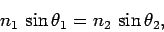(341)

whereis the angle subtended between the incident ray and the normal to the interface, and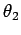is the angle subtended between the refracted ray and the normal to the interface. The quantities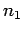and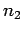are termed the refractive indices of media 1 and 2, respectively. Thus, the law of refraction predicts that a light-ray always deviates more towards the normal in the optically denser medium: i.e., the medium with the higher refractive index. Note that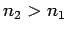in the figure. The law of refraction also holds for non-planar interfaces, provided that the normal to the interface at any given point is understood to be the normal to the local tangent plane of the interface at that point.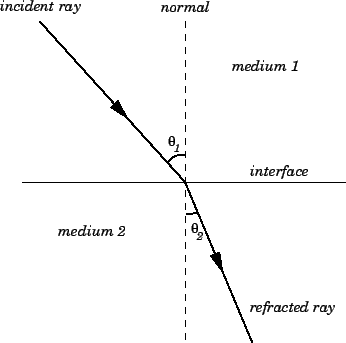By definition, the refractive index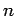of a dielectric medium of dielectric constant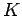is given by(342)

Table 4 shows the refractive indices of some common materials (for yellow light of wavelength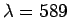nm).

Table 4: Refractive indices of some common materials atnm.
 MaterialAir (STP) 1.00029 Water 1.33 Ice 1.31 Glass: Light flint 1.58 Heavy flint 1.65 Heaviest flint 1.89 Diamond 2.42

The law of refraction follows directly from the fact that the speedwith which light propagates through a dielectric medium is inversely proportional to the refractive index of the medium (see Sect. 11.3). In fact,(343)

whereis the speed of light in a vacuum. Consider two parallel light-rays,and, incident at an anglewith respect to the normal to the interface between two dielectric media, 1 and 2. Let the refractive indices of the two media beandrespectively, with. It is clear from Fig. 58 that raymust move from pointto point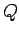, in medium 1, in the same time interval,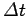, in which raymoves between pointsand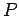, in medium 2. Now, the speed of light in medium 1 is, whereas the speed of light in medium 2 is. It follows that the length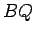is given by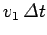, whereas the length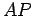is given by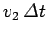. By trigonometry,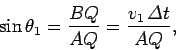(344)

and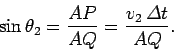(345)

Hence,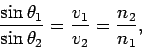(346)

which can be rearranged to give Snell's law. Note that the linesand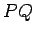represent wave-fronts in media 1 and 2, respectively, and, therefore, cross raysandat right-angles.When light passes from one dielectric medium to another its velocitychanges, but its frequency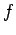remains unchanged. Since,for all waves, where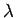is the wavelength, it follows that the wavelength of light must also change as it crosses an interface between two different media. Suppose that light propagates from medium 1 to medium 2. Letandbe the refractive indices of the two media, respectively. The ratio of the wave-lengths in the two media is given by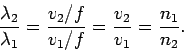(347)

Thus, as light moves from air to glass its wavelength decreases.Next: Total Internal Reflection Up: Geometric Optics Previous: Law of Reflection
Richard Fitzpatrick 2007-07-14